# How to Average Based on Days of Week in Excel?

Consider the following scenario: in a business, you want to know the average sales of weekdays, weekends, and sales on a specific day. This problem can be solved using the formulas provided in Excel. This tutorial will help you understand how we can average based on the day of the week in Excel. The first method is for a specific day of the week, the second is for weekdays, and the third is for weekends.

## Average Based on Specific Days of Week

Here we will use the formula to get the first result, then use the auto-fill handle to get all the results. Let us see a straightforward process to understand how we can average based on specific days of the week in Excel.

Let us assume an Excel sheet that contains a list of dates and sales, as shown in the below image.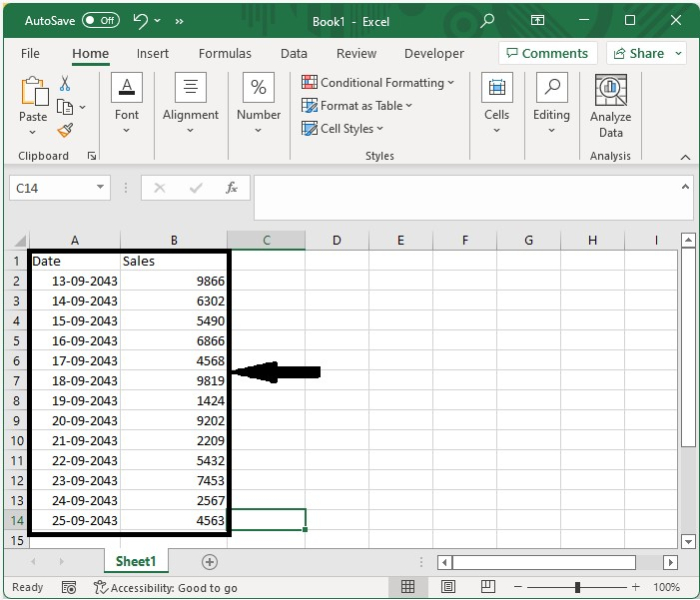Now click on an empty cell and enter the formula as =AVERAGE(IFWEEKDAY(A2:A14)=2,B2:B14)) and press "CTRL+SHIFT+ENTER" to get the formula's first result, "2" (Monday).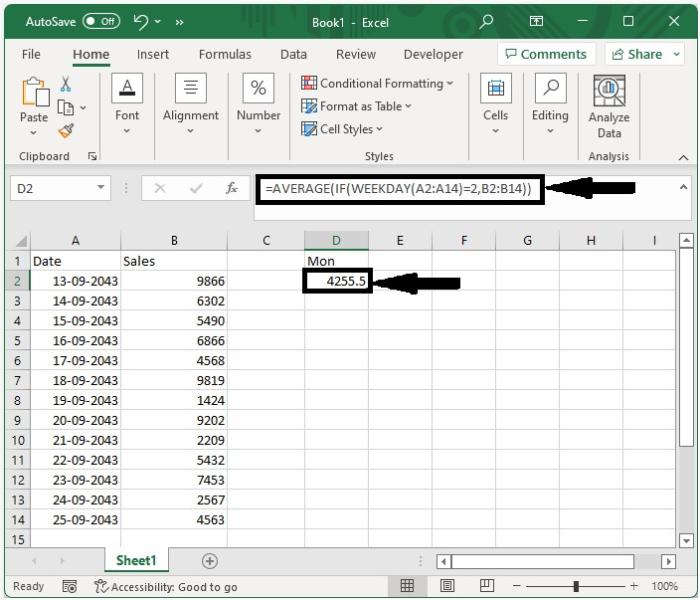## Average Based on Weekdays of Week

Here we will use the formula to get the first result, then use the auto-fill handle to get all the results. Let us see an uncomplicated process to see how we can average based on the days of the week in Excel.

Consider the same data as in the previous example and enter the formula =AVERAGE(IF(WEEKDAY(A2:A14,2)=1,2,3,4,5,B2:B14)) and press "CTRL+SHIFT+ENTER" to get the result shown in the image below.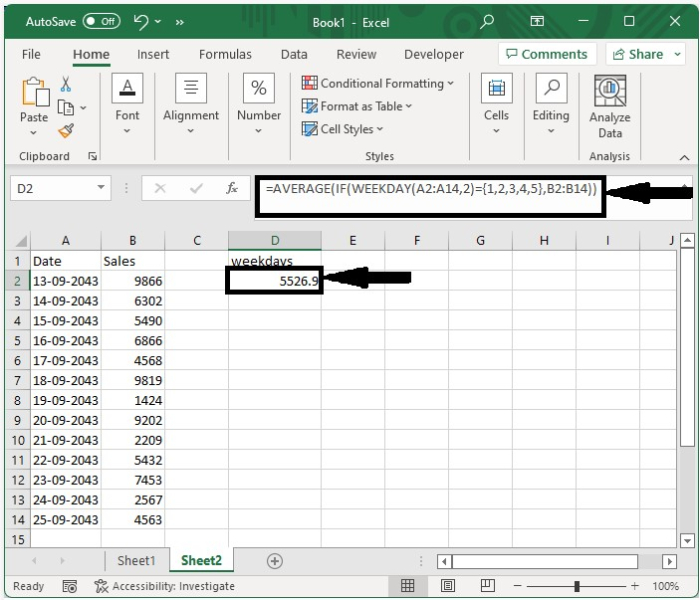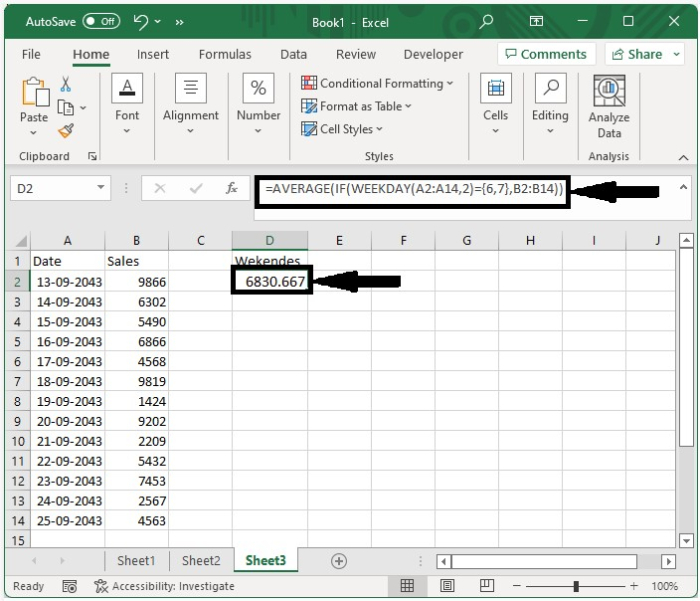## Average Based on Weekends of Week

Here we will use the formula to get the first result, then use the auto-fill handle to get all the results. Let's look at an easy way to see averages based on weekends and weeks in Excel.

Consider the same data as in the previous example and enter the formula =AVERAGE(IF(WEEKDAY(A2:A14,2)=6,7,B2:B14)) and press "CTRL+SHIFT+ENTER" to obtain the result shown in the image below.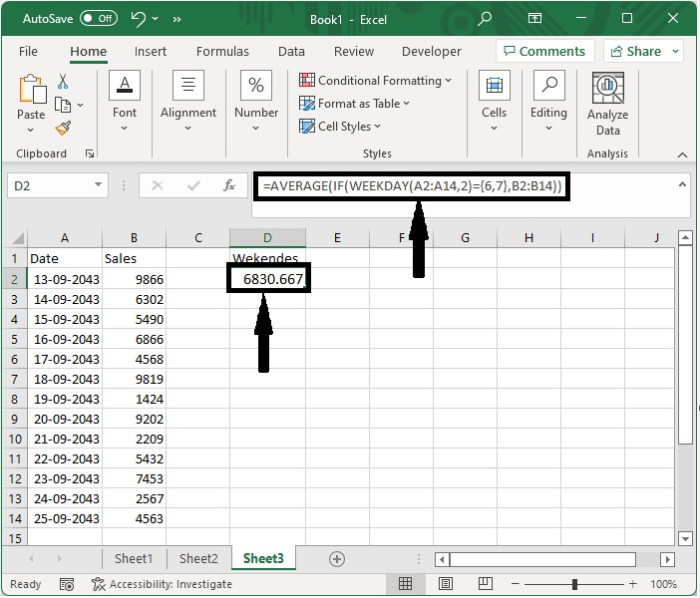We can either enter the result directly or use a formula to get the result.

=SUMPRODUCT((WEEKDAY(A2:A14,2)=1)*B2:B14)/ SUMPRODUCT((WEEKDAY(A2:A14,2)=1)*1)

=SUMPRODUCT((WEEKDAY(A2:A14,2)<6)*B2:B14)/ SUMPRODUCT((WEEKDAY(A2:A14,2)<6)*1)

=SUMPRODUCT((WEEKDAY(A2:A14,2)>5)*B2:B14)/ SUMPRODUCT((WEEKDAY(A2:A14,2)>5)*1).

## Conclusion

In this tutorial, we used a simple example to demonstrate how we can average based on the day of the week in Excel.

Updated on: 12-Jan-2023

2K+ Views